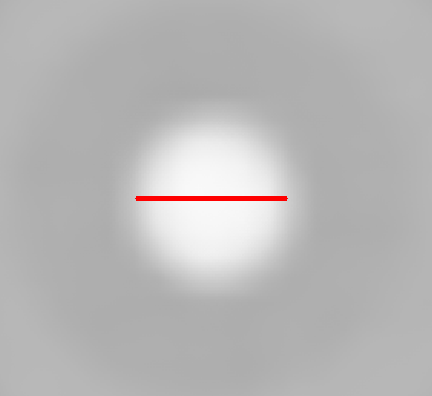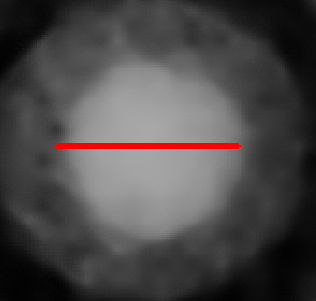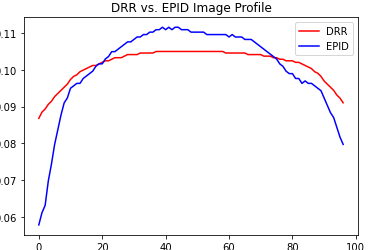# Registration evaluation

Hello! I want to share this problem and ask for some advice. Basically I did a customized registration without using Simple ITK just based on the recognition of the “geometric” circle of the two spheres in the two images below (one is the reference DRR and the other one the Portal Image). The centers of the two spheres (detected circles) are superimposed and I wanted to find a way to evaluate the precision of this overlapping. What I did is drawing the profile of the real diameters of the spheres, normalize them, but I don’t know how much this could be significant since the intensity of the values of the pixel is different (the images are multimodal). I wanted to ask if there’s a way and which is the best to evaluate this registration and how to do it. Thanks in advance.Hello @Enrico_Sarneri,

Basic sanity check:
Compare the similarity metric (mutual information for multi-modal) before and after registration, doesn’t give much just that the registration seems to be going in the right direction (MetricEvaluate).

Quantitative evaluation:

As you are imaging 3D spheres, their 2D perspective projection is a circle.

Single sphere:

1. Estimate the center of the circle from each of the imaging modalities and compare the distance between the two centers. This jupyter notebook illustrates how to localize the center of 3D spheres, modifying it for 2D is trivial.

Multiple spheres:

1. For each circle estimate the center above and compute the distances between corresponding circle centers.
2. Evaluate the registration as described in this jupyter notebook section titled Quantitative evaluation (summary stats, histogram of error distribution and finally view the error distribution in spatial context).
1 Like

Just an idea but maybe some use of PCA could be interesting here